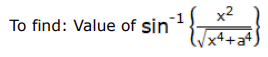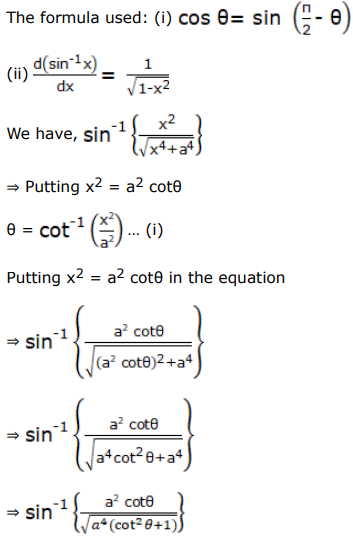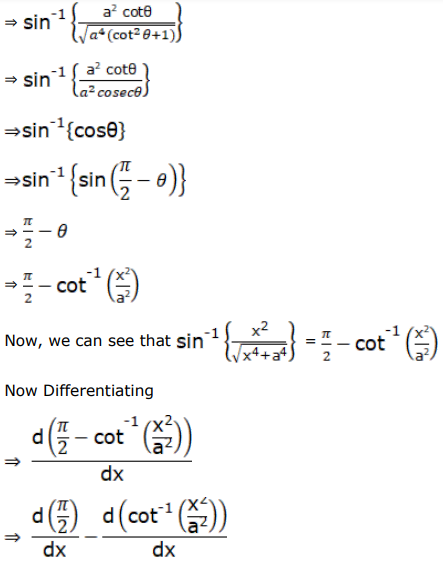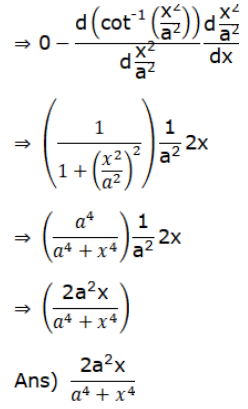# Differentiate each of the following w.r.tQuestion:

Differentiate each of the following w.r.t $x$ :

$\sin ^{-1}\left\{\frac{x^{2}}{\sqrt{x^{4}+a^{4}}}\right\}$

Solution: